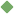gravitational_theory 1. [ noun ] (physics) the theory that any two particles of matter attract one another with a force directly proportional to the product of their masses and inversely proportional to the square of the distance between them Synonyms: theory_of_gravitation theory_of_gravity newton's_theory_of_gravitation Related terms: scientific_theory law_of_gravitation physics To share this definition press "text" (Facebook, Twitter) or "link" (blog, mail) then paste text link Similar spelling: gravitational_force   gravitational_mass   gravitational_field   gravitational_constant   gravitational_collapse